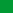Db2 11.5

# CASE expression

CASE expressions allow an expression to be selected based on the evaluation of one or more conditions.

case-expressionsearched-when-clausesimple-when-clauseNotes:
• 1 If the result type of result-expression is a row type, then the syntax represents a row-case-expression and can only be used where a row-expression is allowed.

In general, the value of the case-expression is the value of the result-expression following the first (leftmost) case that evaluates to true. If no case evaluates to true and the ELSE keyword is present then the result is the value of the result-expression or NULL. If no case evaluates to true and the ELSE keyword is not present then the result is NULL. Note that when a case evaluates to unknown (because of NULLs), the case is not true and hence is treated the same way as a case that evaluates to false.

If the CASE expression is in a VALUES clause, an IN predicate, a GROUP BY clause, or an ORDER BY clause, the search-condition in a searched-when-clause cannot be a quantified predicate, IN predicate using a fullselect, or an EXISTS predicate (SQLSTATE 42625).

When using the simple-when-clause, the value of the expression before the first WHEN keyword is tested for equality with the value of the expression following the WHEN keyword. The data type of the expression before the first WHEN keyword must therefore be comparable to the data types of each expression following the WHEN keyword(s). The expression before the first WHEN keyword in a simple-when-clause cannot include a function that is not deterministic or has an external action (SQLSTATE 42845).

A result-expression is an expression following the THEN or ELSE keywords. There must be at least one result-expression in the CASE expression (NULL cannot be specified for every case) (SQLSTATE 42625). All result expressions must have compatible data types (SQLSTATE 42804).

## Examples

• If the first character of a department number is a division in the organization, then a CASE expression can be used to list the full name of the division to which each employee belongs:
``````   SELECT EMPNO, LASTNAME,
CASE SUBSTR(WORKDEPT,1,1)
WHEN 'B' THEN 'Human Resources'
WHEN 'C' THEN 'Accounting'
WHEN 'D' THEN 'Design'
WHEN 'E' THEN 'Operations'
END
FROM EMPLOYEE;``````
• The number of years of education are used in the EMPLOYEE table to give the education level. A CASE expression can be used to group these and to show the level of education.
``````   SELECT EMPNO, FIRSTNME, MIDINIT, LASTNAME,
CASE
WHEN EDLEVEL < 15 THEN 'SECONDARY'
WHEN EDLEVEL < 19 THEN 'COLLEGE'
END
FROM EMPLOYEE``````
• Another interesting example of CASE statement usage is in protecting from division by 0 errors. For example, the following code finds the employees who earn more than 25% of their income from commission, but who are not fully paid on commission:
``````   SELECT EMPNO, WORKDEPT, SALARY+COMM FROM EMPLOYEE
WHERE (CASE WHEN SALARY=0 THEN NULL
ELSE COMM/SALARY
END) > 0.25;``````
• The following CASE expressions are the same:
``````   SELECT LASTNAME,
CASE
WHEN LASTNAME = 'Haas' THEN 'President'
...

SELECT LASTNAME,
CASE LASTNAME
WHEN 'Haas' THEN 'President'
...``````

There are two scalar functions, NULLIF and COALESCE, that are specialized to handle a subset of the functionality provided by CASE. Table 1 shows the equivalent expressions using CASE or these functions.

Table 1. Equivalent CASE Expressions
Expression Equivalent Expression
``````CASE
WHEN e1=e2 THEN NULL
ELSE e1
END``````
NULLIF(e1,e2)
``````CASE
WHEN e1 IS NOT NULL THEN e1
ELSE e2
END``````
COALESCE(e1,e2)
``````CASE
WHEN e1 IS NOT NULL THEN e1
ELSE COALESCE(e2,...,eN)
END``````
COALESCE(e1,e2,...,eN)
``````CASE
WHEN c1=var1 OR (c1 IS NULL AND var1 IS NULL)
THEN 'a'
WHEN c1=var2 OR (c1 IS NULL AND var2 IS NULL)
THEN 'b'
ELSE NULL
END``````
DECODE(c1,var1, 'a', var2, 'b')